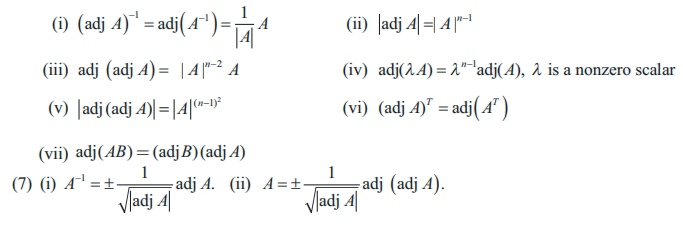Home | | Maths 12th Std | Summary

# Summary

Mathematics : Applications of Matrices and Determinants: Summary

SUMMARY

(1) Adjoint of a square matrix A = Transpose of the cofactor matrix of A .

(3) A-1 = [ 1/|A| ] adj A.

(4)where ╬╗ is a non-zero scalar.

(5) (i) (AB)-1 = B-1 A-1 . (ii) ((A)-1 )-1=A

(6) If A is a non-singular square matrix of order n , then(8) (i) A matrix A is orthogonal if AAT = AT A = I

(ii) A matrix A is orthogonal if and only if A is non-singular and AÔłĺ1 = AT

(8) Methods to solve the system of linear equations AX = B

(i) By matrix inversion method X = A-1B, | A | Ôëá 0

(ii) (ii) By CramerÔÇÖs rule(iii) By Gaussian elimination method

(9) (i) If ¤ü ( A) = ¤ü ([ A | B]) = number of unknowns, then the system has unique solution.

(ii) If ¤ü ( A) = ¤ü ([ A | B]) <  number of unknowns, then the system has infinitely many solutions.

(iii) If ¤ü ( A) Ôëá ¤ü ([ A | B]) then the system is inconsistent and has no solution.

(10) The homogenous system of linear equations AX = 0

(i) has the trivial solution, if | A | Ôëá 0 .

(ii) has a non trivial solution, if | A |= 0 .

Tags : Applications of Matrices and Determinants , 12th Mathematics : UNIT 1 : Applications of Matrices and Determinants
Study Material, Lecturing Notes, Assignment, Reference, Wiki description explanation, brief detail
12th Mathematics : UNIT 1 : Applications of Matrices and Determinants : Summary | Applications of Matrices and Determinants